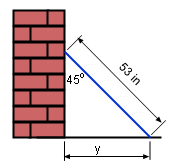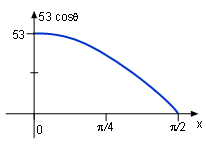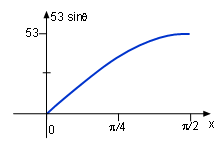Ch 2. Derivatives I Multimedia Engineering Math DerivativeFormulas TrigonometricFunctions Differentiability ChainRule ImplicitDifferentiation
 Chapter 1. Limits 2. Derivatives I 3. Derivatives II 4. Mean Value 5. Curve Sketching 6. Integrals 7. Inverse Functions 8. Integration Tech. 9. Integrate App. 10. Parametric Eqs. 11. Polar Coord. 12. Series Appendix Basic Math Units Search eBooks Dynamics Fluids Math Mechanics Statics Thermodynamics Author(s): Hengzhong Wen Chean Chin Ngo Meirong Huang Kurt Gramoll ©Kurt GramollMATHEMATICS - CASE STUDY SOLUTIONTilt ice Hockey Stick A 53 in ice hockey stick rests against a vertical wall. The bottom of the stick slides away from the wall. How fast does the distance, y change with respect to the angle, θ, which is made by the wall and the stick, when θ is equals 45o? Assumption: The angle made by the wall and the stick is θ. The slipped distance is y.y = 53 sinθdy/dx = 53 cosθ According to the diagram,      sinθ = y/53 Thus      y = 53 sinθ Derivative y with respect to θ      dy/dθ = d(53 sinθ)/dθ               =53 cosθ When θ = 45o,      dy/dθ = 53 cosθ = 37.5 in/rad The derivative of y = 53 sinθ is dy/dθ = 53 cosθ when θ is in the range of (0, π/2). Since y is differentiable, it is continuous, this is shown in the plot of y = 53 sinθ.